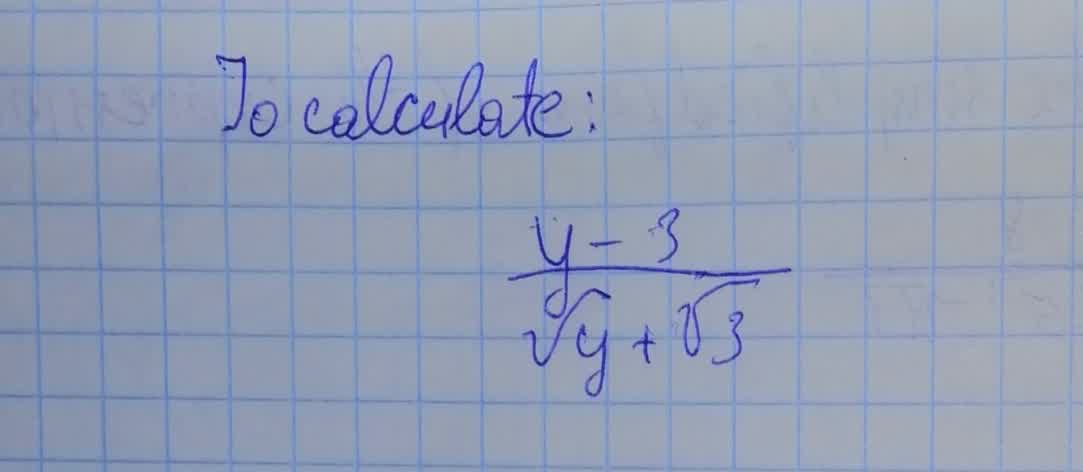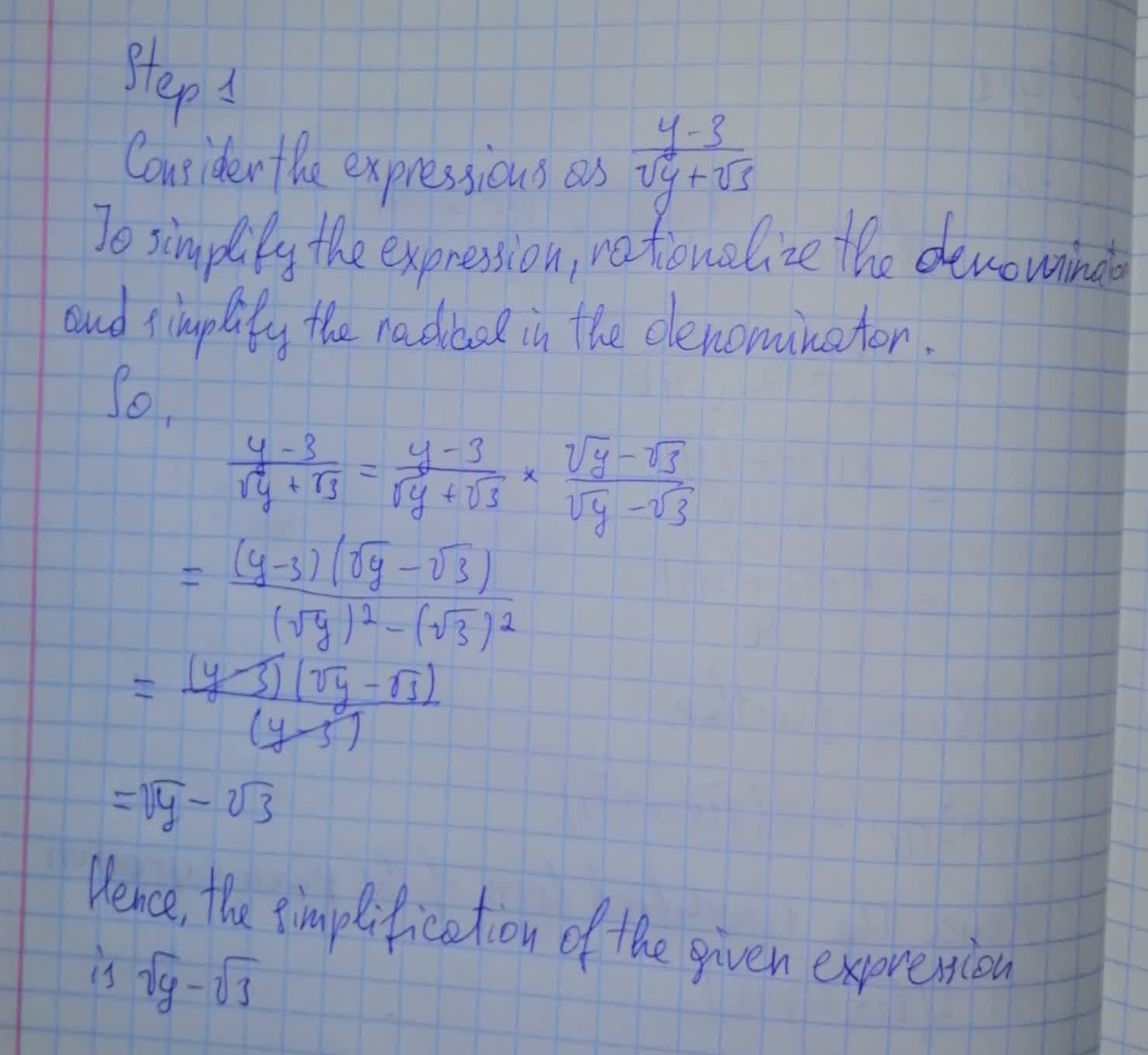To calculate: The simplification of the expression \frac{y-3}{\sqrt{y}+\sqrt{3}}Tammy Todd 2021-08-14 Answered
To calculate: The simplification of the expression $$\displaystyle{\frac{{{y}-{3}}}{{\sqrt{{{y}}}+\sqrt{{{3}}}}}}$$• Questions are typically answered in as fast as 30 minutes

Solve your problem for the price of one coffee

• Math expert for every subject
• Pay only if we can solve itDemi-Leigh Barrera
Calculation:Not exactly what you’re looking for?

Step 1

Consider the expressions as,

$$\frac{y-3}{\sqrt y+\sqrt3}$$

To simplify the expression, rationalise the denomination and simplify the radical in the denomination

So,

$$\frac{y-3}{\sqrt y+\sqrt3}=\frac{y-3}{\sqrt y+\sqrt 3} \times \frac{\sqrt y-\sqrt 3}{\sqrt y-\sqrt 3}$$

$$=\frac{(y-3)(\sqrt y-\sqrt3)}{(\sqrt y)^2-(\sqrt 3)^2}$$

$$=\frac{(y-3)(\sqrt y-\sqrt 3)}{(y-3)}$$

$$=\sqrt y-\sqrt3$$

Hence, the simplification of the given expression is $$\sqrt y-\sqrt3$$